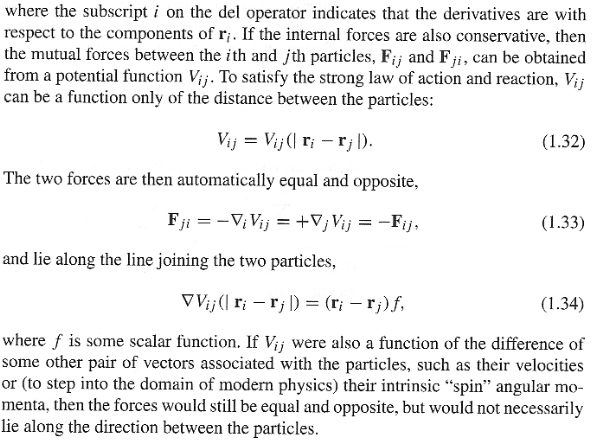# Prove forces derived from a velocity-dependent potential are not central

I don't see why the last sentence in the attachment is true. It claims that if ##V_{ij}## or simply ##V## is also a function of the difference of velocities of particles ##i## and ##j##, then the force derived from ##V## is not central. In other words, if ##V=V(|r_i-r_j|, |v_i-v_j|)##, then (1.34) is not satisfied.

Let ##p=|r_i-r_j|## and ##q=|v_i-v_j|## and ##\partial_x=\frac{\partial}{\partial x}##.

##\nabla_iV(p, q)=\partial_{x_i}V(p, q)\vec{e_x}+\partial_{y_i}V(p, q)\vec{e_y}+\partial_{z_i}V(p, q)\vec{e_z}##. Definition of ##\nabla_i## is given by the first sentence of the attachment.

Since ##\partial_{x_i}V(p, q)=\partial_{x_i}V(p)##, the RHS above should be the same as the RHS of (1.34). Then, (1.34) will be satisfied.

##\partial_{x_i}V(p, q)=\partial_{x_i}V(p)## for the same reason as ##\partial_x(x+\dot{x}^2)=1=\partial_xx##.EDIT: I found the mistake. ##\partial_{x_i}V(p, q)\neq\partial_{x_i}V(p)## for the same reason as ##\partial_x(x+x\dot{x})\neq\partial_xx##.

Last edited:

Nonetheless, I am still able to prove that the force derived from ##V=V(p, q)## satisfies (1.34), contrary to what is claimed by the book.

Recall that ##p=|r_i-r_j|=\sqrt{(x_i-x_j)^2+(y_i-y_j)^2+(z_i-z_j)^2}## and ##q=|v_i-v_j|##.

Thus, ##\frac{\partial p}{\partial x_i}=\frac{1}{2p}2(x_i-x_j)=\frac{1}{p}(x_i-x_j)##.

Let ##V'(p, q)=\partial_{p}V(p, q)##.

##\nabla_iV(p, q)=\partial_{x_i}V(p, q)\vec{e_x}+\partial_{y_i}V(p, q)\vec{e_y}+\partial_{z_i}V(p, q)\vec{e_z}##

##=V'(p, q)\frac{\partial p}{\partial x_i}\vec{e_x}+V'(p, q)\frac{\partial p}{\partial y_i}\vec{e_y}+V'(p, q)\frac{\partial p}{\partial z_i}\vec{e_z}##

##=\frac{V'(p, q)}{p}(x_i-x_j)\vec{e_x}+\frac{V'(p, q)}{p}(y_i-y_j)\vec{e_y}+\frac{V'(p, q)}{p}(z_i-z_j)\vec{e_z}##

##=\frac{V'(p, q)}{p}(\vec{r_i}-\vec{r_j})##,

which is equivalent to the RHS of (1.34).

What's wrong?

Last edited: